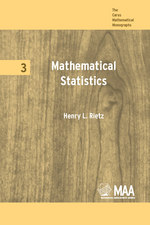Have library access? Log in through your library# Mathematical Statistics

HENRY LEWIS RIETZ
Volume: 3
Copyright Date: 1927
Edition: 1
Pages: 194
https://www.jstor.org/stable/10.4169/j.ctt5hh89g

## Table of Contents

1. Front Matter
(pp. i-iv)
2. PREFACE
(pp. v-viii)
Henry L. Rietz
3. Table of Contents
(pp. ix-xii)
4. CHAPTER I THE NATURE OF THE PROBLEMS AND UNDERLYING CONCEPTS OF MATHEMATICAL STATISTICS
(pp. 1-21)

The bounds of mathematical statistics are not sharply denned. It is not uncommon to include under mathematical statistics such topics as interpolation theory, approximate integration, periodogram analysis, index numbers, actuarial theory, and various other topics from the calculus of observations. In fact, it seems that mathematical statistics in its most extended meaning may be regarded as including all the mathematics applied to the analysis of quantitative data obtained from observation. On the other hand, a number of mathematicians and statisticians have implied by their writings a limitation of mathematical statistics to the consideration of such questions of frequency, probability, averages,...

5. CHAPTER II RELATIVE FREQUENCIES IN SIMPLE SAMPLING
(pp. 22-45)

In Chapter I attention was directed to the very simple process of finding the relative frequency of occurrence of an event or character among s cases in question. Let us now conceive of repeating the process of finding relative frequencies on many random samples each consisting of s items drawn from the same population. To characterize the degree of stability or the degree of dispersion of such a series of relative frequencies is a fundamental statistical problem.

To illustrate, suppose we repeat the throwing of a set of 1,000 coins many times. An observed frequency distribution could then be exhibited...

6. CHAPTER III FREQUENCY FUNCTIONS OF ONE VARIABLE
(pp. 46-76)

In Chapter I we have discussed very briefly three different methods of describing frequency distributions of one variable—the purely graphic method, the method of averages and measures of dispersion, and the method of theoretical frequency functions or curves. The weakness and inadequacy of the purely graphic method lies in the fact that it fails to give a numerical description of the distribution. While the method of averages and measures of dispersion gives a numerical description in the form of a summary characterization which is likely to be useful for many statistical purposes, particularly for purposes of comparison, the method...

7. CHAPTER IV CORRELATION
(pp. 77-113)

Suppose we have data consisting ofNpairs of corresponding variates (xi,yi),i= 1, 2, . . . . ,N. The given pairs of values may arise from any one of a great variety of situations. For example, we may have a group of men in whichxrepresents the height of a man andyhis weight; we may have a group of fathers and their oldest sons in whichxis the stature of a father andythat of his oldest son; we may have minimal daily temperatures in whichxis the minimal daily...

8. CHAPTER V On Random Sampling Fluctuations
(pp. 114-145)

In Chapter II we have dealt to some extent with the effects of random sampling fluctuations on relative frequencies. But it is fairly obvious that the interest of the statistician in the effects of sampling fluctuations extends far beyond the fluctuations in relative frequencies. To illustrate, suppose we calculate any statistical measure such as an arithmetic mean, median, standard deviation, correlation coefficient, or parameter of a frequency function from the actual frequencies given by a sample of data. If we need then either to form a judgment as to the stability of such results from sample to sample or to...

9. CHAPTER VI THE LEXIS THEORY
(pp. 146-155)

We have throughout Chapter II assumed a constant probability underlying, the frequency ratios obtained from observation. It is fairly obvious that frequency ratios are often found from material in which the underlying probability is not constant. Then the statistician should make use of all available knowledge of the material for appropriate classification into subsets for analysis and comparison. It thus becomes important to consider a set of observations which may be broken into subsets for examination and comparison as to whether the underlying probability seems to be constant from subset to subset. In the separation of a large number of...

10. CHAPTER VII A DEVELOPMENT OF THE GRAM-CHARLIER SERIES
(pp. 156-172)

In § 56 we shall attempt to show (cf. p. 65) that a certain line of development²⁴ of the binomial distribution suggests the use of the Gram-Charlier Type A series as a natural extension of the De Moivre-Laplace approximation and the Type B series as a natural extension of the Poisson exponential approximation considered in Chapter II. Then in §§ 57–58 we shall develop methods for the determination of the parameters in terms of moments of the observed frequency distribution, thus deriving certain results stated without proof in § 19 and § 21.

As in the De Moivre-Laplace theory,...

11. NOTES
(pp. 173-178)
12. INDEX
(pp. 179-181)